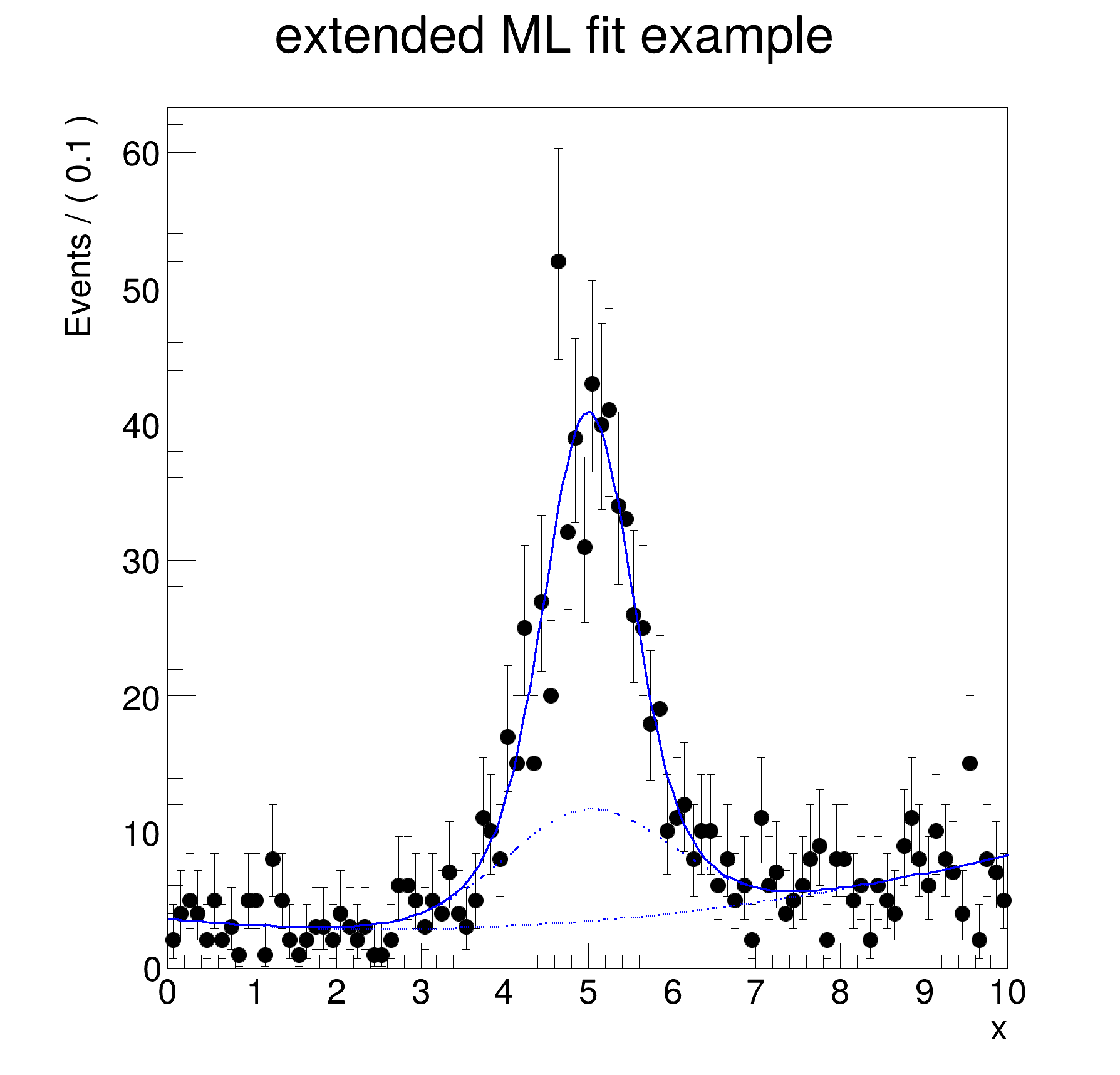ROOT   Reference Guide
Searching...
No Matches
rf202_extendedmlfit.py File Reference

## Namespaces

namespace  rf202_extendedmlfit

## Detailed DescriptionAddition and convolution: setting up an extended maximum likelihood fitimport ROOT
# Set up component pdfs
# ---------------------------------------
# Declare observable x
x = ROOT.RooRealVar("x", "x", 0, 10)
# Create two Gaussian PDFs g1(x,mean1,sigma) anf g2(x,mean2,sigma) and
# their parameters
mean = ROOT.RooRealVar("mean", "mean of gaussians", 5)
sigma1 = ROOT.RooRealVar("sigma1", "width of gaussians", 0.5)
sigma2 = ROOT.RooRealVar("sigma2", "width of gaussians", 1)
sig1 = ROOT.RooGaussian("sig1", "Signal component 1", x, mean, sigma1)
sig2 = ROOT.RooGaussian("sig2", "Signal component 2", x, mean, sigma2)
# Build Chebychev polynomial pdf
a0 = ROOT.RooRealVar("a0", "a0", 0.5, 0.0, 1.0)
a1 = ROOT.RooRealVar("a1", "a1", -0.2, 0.0, 1.0)
bkg = ROOT.RooChebychev("bkg", "Background", x, [a0, a1])
# Sum the signal components into a composite signal pdf
sig1frac = ROOT.RooRealVar("sig1frac", "fraction of component 1 in signal", 0.8, 0.0, 1.0)
sig = ROOT.RooAddPdf("sig", "Signal", [sig1, sig2], [sig1frac])
# Method 1 - Construct extended composite model
# -------------------------------------------------------------------
# Sum the composite signal and background into an extended pdf
# nsig*sig+nbkg*bkg
nsig = ROOT.RooRealVar("nsig", "number of signal events", 500, 0.0, 10000)
nbkg = ROOT.RooRealVar("nbkg", "number of background events", 500, 0, 10000)
model = ROOT.RooAddPdf("model", "(g1+g2)+a", [bkg, sig], [nbkg, nsig])
# Sample, fit and plot extended model
# ---------------------------------------------------------------------
# Generate a data sample of expected number events in x from model
# = model.expectedEvents() = nsig+nbkg
data = model.generate({x})
# Fit model to data, ML term automatically included
model.fitTo(data, PrintLevel=-1)
# Plot data and PDF overlaid, expected number of events for pdf projection normalization
# rather than observed number of events (==data.numEntries())
xframe = x.frame(Title="extended ML fit example")
data.plotOn(xframe)
model.plotOn(xframe, Normalization=dict(scaleFactor=1.0, scaleType=ROOT.RooAbsReal.RelativeExpected))
# Overlay the background component of model with a dashed line
model.plotOn(
xframe,
Components={bkg},
LineStyle=":",
Normalization=dict(scaleFactor=1.0, scaleType=ROOT.RooAbsReal.RelativeExpected),
)
# Overlay the background+sig2 components of model with a dotted line
ras_bkg_sig2 = {bkg, sig2}
model.plotOn(
xframe,
Components=ras_bkg_sig2,
LineStyle=":",
Normalization=dict(scaleFactor=1.0, scaleType=ROOT.RooAbsReal.RelativeExpected),
)
# Print structure of composite pdf
model.Print("t")
# Method 2 - Construct extended components first
# ---------------------------------------------------------------------
# Associated nsig/nbkg as expected number of events with sig/bkg
esig = ROOT.RooExtendPdf("esig", "extended signal pdf", sig, nsig)
ebkg = ROOT.RooExtendPdf("ebkg", "extended background pdf", bkg, nbkg)
# Sum extended components without coefs
# -------------------------------------------------------------------------
# Construct sum of two extended pdf (no coefficients required)
model2 = ROOT.RooAddPdf("model2", "(g1+g2)+a", [ebkg, esig])
# Draw the frame on the canvas
c = ROOT.TCanvas("rf202_extendedmlfit", "rf202_extendedmlfit", 600, 600)
xframe.GetYaxis().SetTitleOffset(1.4)
xframe.Draw()
c.SaveAs("rf202_extendedmlfit.png")
[#0] WARNING:InputArguments -- The parameter 'sigma1' with range [-inf, inf] of the RooGaussian 'sig1' exceeds the safe range of (0, inf). Advise to limit its range.
[#0] WARNING:InputArguments -- The parameter 'sigma2' with range [-inf, inf] of the RooGaussian 'sig2' exceeds the safe range of (0, inf). Advise to limit its range.
[#1] INFO:Minimization -- p.d.f. provides expected number of events, including extended term in likelihood.
[#1] INFO:Minimization -- RooAbsMinimizerFcn::setOptimizeConst: activating const optimization
[#1] INFO:Minimization -- The following expressions have been identified as constant and will be precalculated and cached: (sig1,sig2)
[#1] INFO:Minimization -- The following expressions will be evaluated in cache-and-track mode: (bkg)
[#1] INFO:Minimization -- RooAbsMinimizerFcn::setOptimizeConst: deactivating const optimization
[#1] INFO:Plotting -- RooAbsPdf::plotOn(model) directly selected PDF components: (bkg)
[#1] INFO:Plotting -- RooAbsPdf::plotOn(model) indirectly selected PDF components: ()
[#1] INFO:Plotting -- RooAbsPdf::plotOn(model) directly selected PDF components: (bkg,sig2)
[#1] INFO:Plotting -- RooAbsPdf::plotOn(model) indirectly selected PDF components: (sig)
0x7f5d8c0/V- RooChebychev::bkg = 0.733482 [Auto,Dirty]
0x7a8c370/V- RooRealVar::x = 5
0x14f7720/V- RooRealVar::a0 = 0.507382 +/- 0.0795949
0x7bce0f0/V- RooRealVar::a1 = 0.266518 +/- 0.133887
0x809d5c0/V- RooRealVar::nbkg = 427.704 +/- 38.0643
0x7c06910/V- RooGaussian::sig1 = 1 [Auto,Dirty]
0x7a8c370/V- RooRealVar::x = 5
0x2c2d610/V- RooRealVar::mean = 5
0x2a22ac0/V- RooRealVar::sigma1 = 0.5
0x7e6f690/V- RooRealVar::sig1frac = 0.640056 +/- 0.0966619
0x7bbab90/V- RooGaussian::sig2 = 1 [Auto,Dirty]
0x7a8c370/V- RooRealVar::x = 5
0x2c2d610/V- RooRealVar::mean = 5
0x1528020/V- RooRealVar::sigma2 = 1
0x7ea1850/V- RooRealVar::nsig = 572.124 +/- 39.9094
Date
February 2018

Definition in file rf202_extendedmlfit.py.